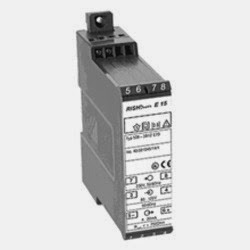# Loop Resistance in Current Signal Circuits

Current Signal have become extremely popular in recent decades.  Current signals have an inherent resistance to noise.  Current signals can also travel over long distances as compared to voltage signals.  Loop resistance is an important factor in current signals.In current signals, the sensor varies its resistance depending on the parameter to be measured.  This varies the current.  The maximum current the source (usually the recording or measuring instrument) can drive is depending on the total resistance of the circuit.  The total resistance of the current signal circuit depends on the resistance of the sensor and the wire resistance.

Each channel of the analog measuring or recording instrument (PLC or any recorder) has a maximum resistance across which it can drive the resistance. Hence, it is necessary to ensure that the total resistance does not exceed this resistance.

The wire resistance can be measured by shorting the wires at one end and measuring the resistance from the other end using a multimeter.  This resistance has to be added to the sensor resistance (usually mentioned in the datasheet of the sensor) to give the total resistance.

Sometimes, over the course of many years, a new meter may be connected in series to the circuit.  This may increase the loop resistance and the value will be affected.  Hence, whenever any new instrument is added to the current signal circuit, the resistance needs to be recalculated.